# Binomial Distribution

• BINP

• B = Binary process = 2 process

• I = Independent event

• N = Number of trials

• P = Probability of success

• Binomial Probability

• binompdf(n, p, x)

• n=trials

• p=probability

• x=value• Calculator

• 2ND + VARS (DISTR)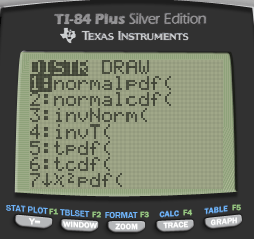• A: binompdf / B: binomcdf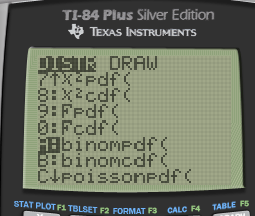• binompdf vs binomcdf# Practice Questions for Binomial Distribution

• A manufacturer produces a large number of toasters. From past experience, the manufacturer knows that approximately 4% are defective. In a quality control procedure, we randomly select 40 toasters for testing.

• Determine the probability that exactly one of the toasters is defective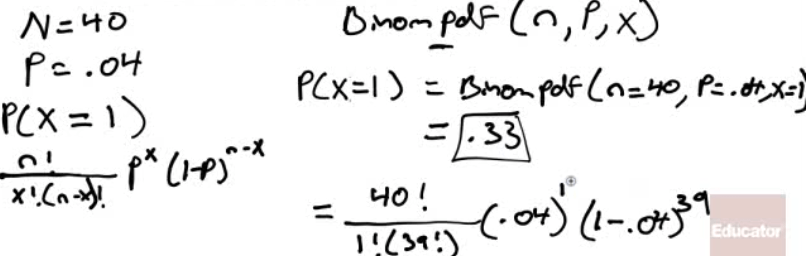• Find the probability that at most two of the toasters are defective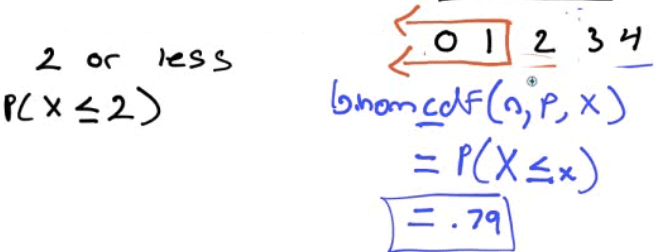• Find the probability that more than three toasters are defective# Geometric Distribution

• BINP

• Not given the number of trials
• Question Format

• How many trials until a success
• Geometric Probability

• geometpdf(p,x)

• geometcdf(p,x)

• p=probability of success

• x=number of trials until 1 success# Practice Questions for Geometric Distribution

• There is a probability of 0.09 that a vaccine will cause a certain side effect. Suppose that a number of patients are inoculated with the vaccine. We are interested in the number of patients vaccinated until the first side effect is observed

• Find the probability that exactly 4 patients must be vaccinated in order to observe the first side effect.

![Т1-84 PIus S'tver Editj(n ф TEns [NSTRUMENTS ](./media/image125.png)• What is the probability that the number of patients vaccinated until the first side effect is observed at most 5?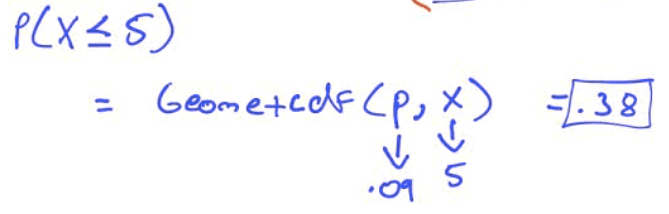# Mean and Standard Deviation